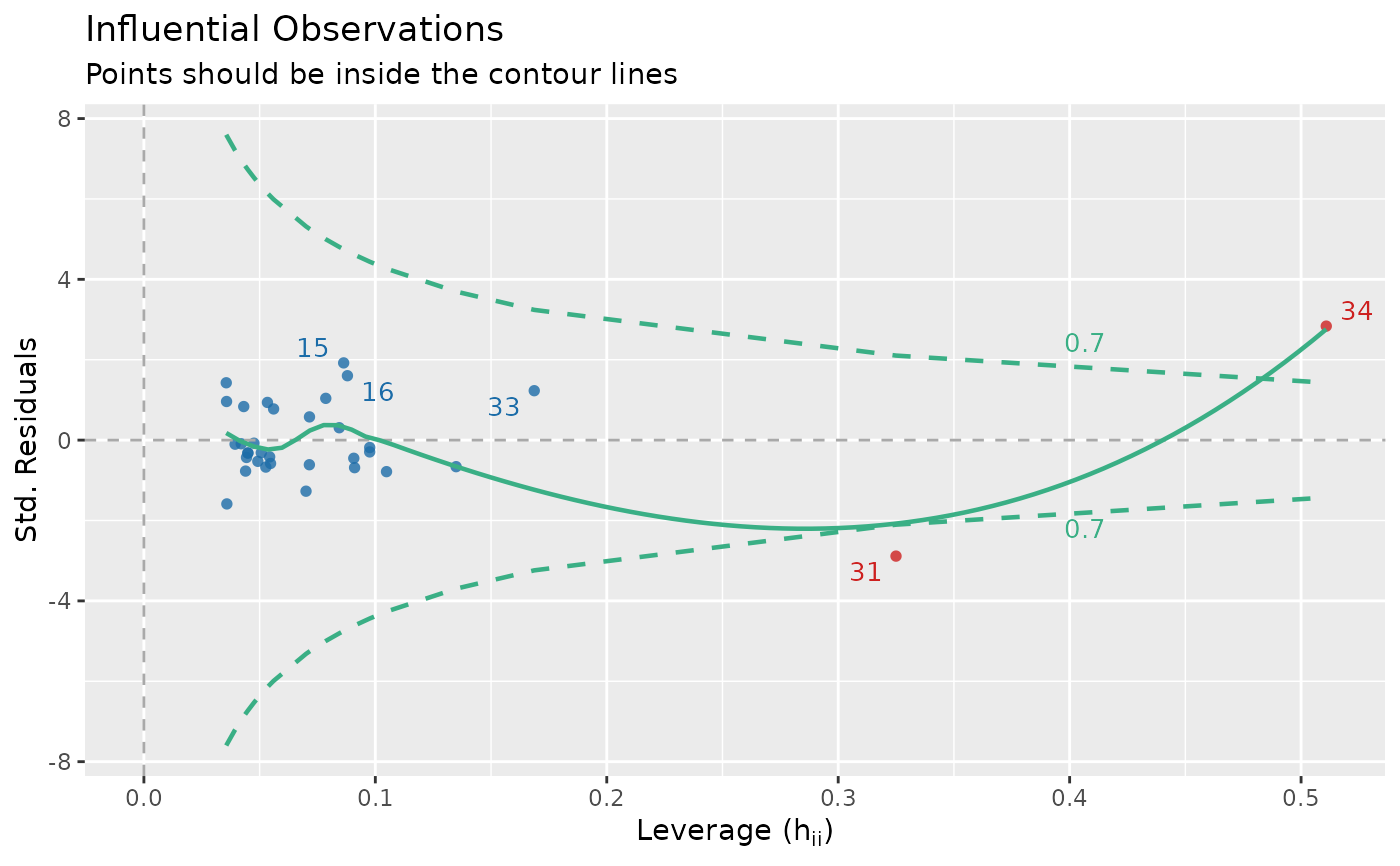The plot() method for the performance::check_outliers() function.

## Usage

# S3 method for see_check_outliers
plot(
x,
size_text = 3.5,
size_line = 0.8,
dot_alpha = 0.8,
colors = c("#3aaf85", "#1b6ca8", "#cd201f"),
rescale_distance = TRUE,
type = c("dots", "bars"),
show_labels = TRUE,
...
)

## Arguments

x

An object.

size_text

Numeric value specifying size of text labels.

size_line

Numeric value specifying size of line geoms.

dot_alpha

Numeric value specifying alpha level of the point geoms.

colors

Character vector of length two, indicating the colors (in hex-format) for points and line.

rescale_distance

Logical. If TRUE, distance values are rescaled to a range from 0 to 1. This is mainly due to better catch the differences between distance values.

type

Character vector, indicating the type of plot.

show_labels

Logical. If TRUE, text labels are displayed.

...

Arguments passed to or from other methods.

## Value

A ggplot2-object.

## Examples

library(performance)
data(mtcars)
mt1 <- mtcars[, c(1, 3, 4)]
mt2 <- rbind(
mt1,
data.frame(mpg = c(37, 40), disp = c(300, 400), hp = c(110, 120))
)
model <- lm(disp ~ mpg + hp, data = mt2)
plot(check_outliers(model))